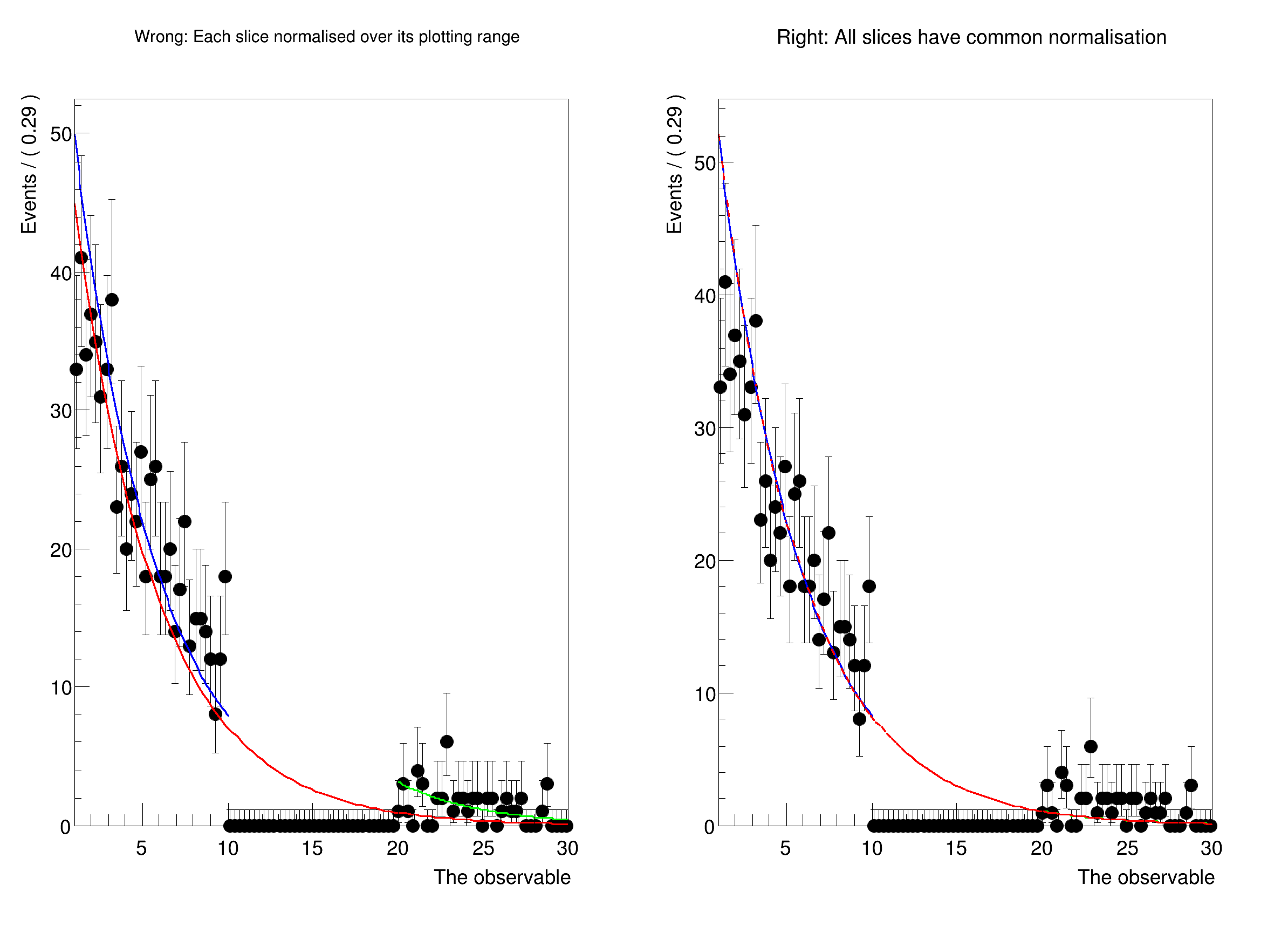ROOT   Reference Guiderf212_plottingInRanges_blinding.C File Reference

## Detailed Description

Plot a PDF in disjunct ranges, and get normalisation right.

Usually, when comparing a fit to data, one should first plot the data, and then the PDF. In this case, the PDF is automatically normalised to match the number of data events in the plot. However, when plotting only a sub-range, when e.g. a signal region has to be blinded, one has to exclude the blinded region from the computation of the normalisation.

In this tutorial, we show how to explicitly choose the normalisation when plotting using NormRange().

Thanks to Marc Escalier for asking how to do this correctly.#include <RooDataSet.h>
#include <RooExponential.h>
#include <RooPlot.h>
#include <RooRealVar.h>
#include <TCanvas.h>
using namespace RooFit;
void rf212_plottingInRanges_blinding()
{
// Make a fit model
RooRealVar x("x", "The observable", 1, 30);
RooRealVar tau("tau", "The exponent", -0.1337, -10., -0.1);
RooExponential exp("exp", "A falling exponential function", x, tau);
// Define the sidebands (e.g. background regions)
x.setRange("full", 1, 30);
x.setRange("left", 1, 10);
x.setRange("right", 20, 30);
// Generate toy data, and cut out the blinded region.
RooDataSet* data = exp.generate(x, 1000);
auto blindedData = data->reduce(CutRange("left,right"));
// Kick tau a bit, and run an unbinned fit where the blinded data are missing.
// ----------------------------------------------------------------------------------------------------------
tau.setVal(-2.);
exp.fitTo(*blindedData);
// Here we will plot the results
TCanvas *canvas=new TCanvas("canvas","canvas",800,600);
canvas->Divide(2,1);
// Wrong:
// Plotting each slice on its own normalises the PDF over its plotting range. For the full curve, that means
// that the blinded region where data is missing is included in the normalisation calculation. The PDF therefore
// comes out too low, and doesn't match up with the slices in the side bands, which are normalised to "their" data.
// ----------------------------------------------------------------------------------------------------------
std::cout << "Now plotting with unique normalisation for each slice." << std::endl;
canvas->cd(1);
RooPlot* plotFrame = x.frame(RooFit::Title("Wrong: Each slice normalised over its plotting range"));
// Plot only the blinded data, and then plot the PDF over the full range as well as both sidebands
blindedData->plotOn(plotFrame);
exp.plotOn(plotFrame, LineColor(kRed), Range("full"));
exp.plotOn(plotFrame, LineColor(kBlue), Range("left"));
exp.plotOn(plotFrame, LineColor(kGreen), Range("right"));
plotFrame->Draw();
// Right:
// Make the same plot, but normalise each piece with respect to the regions "left" AND "right". This requires setting
// a "NormRange", which tells RooFit over which range the PDF has to be integrated to normalise.
// This is means that the normalisation of the blue and green curves is slightly different from the left plot,
// because they get a common scale factor.
// ----------------------------------------------------------------------------------------------------------
std::cout << "\n\nNow plotting with correct norm ranges:" << std::endl;
canvas->cd(2);
RooPlot* plotFrameWithNormRange = x.frame(RooFit::Title("Right: All slices have common normalisation"));
// Plot only the blinded data, and then plot the PDF over the full range as well as both sidebands
blindedData->plotOn(plotFrameWithNormRange);
exp.plotOn(plotFrameWithNormRange, LineColor(kBlue), Range("left"), RooFit::NormRange("left,right"));
exp.plotOn(plotFrameWithNormRange, LineColor(kGreen), Range("right"), RooFit::NormRange("left,right"));
exp.plotOn(plotFrameWithNormRange, LineColor(kRed), Range("full"), RooFit::NormRange("left,right"), LineStyle(10));
plotFrameWithNormRange->Draw();
canvas->Draw();
}
[1mRooFit v3.60 -- Developed by Wouter Verkerke and David Kirkby[0m
Copyright (C) 2000-2013 NIKHEF, University of California & Stanford University
[#1] INFO:Eval -- RooRealVar::setRange(x) new range named 'full' created with bounds [1,30]
[#1] INFO:Eval -- RooRealVar::setRange(x) new range named 'left' created with bounds [1,10]
[#1] INFO:Eval -- RooRealVar::setRange(x) new range named 'right' created with bounds [20,30]
[#1] INFO:Minization -- RooMinimizer::optimizeConst: activating const optimization
**********
** 1 **SET PRINT 1
**********
**********
**********
PARAMETER DEFINITIONS:
NO. NAME VALUE STEP SIZE LIMITS
1 tau -2.00000e+00 9.50000e-01 -1.00000e+01 -1.00000e-01
**********
** 3 **SET ERR 0.5
**********
**********
** 4 **SET PRINT 1
**********
**********
** 5 **SET STR 1
**********
NOW USING STRATEGY 1: TRY TO BALANCE SPEED AGAINST RELIABILITY
**********
**********
FIRST CALL TO USER FUNCTION AT NEW START POINT, WITH IFLAG=4.
START MIGRAD MINIMIZATION. STRATEGY 1. CONVERGENCE WHEN EDM .LT. 1.00e-03
FCN=6752.91 FROM MIGRAD STATUS=INITIATE 4 CALLS 5 TOTAL
EDM= unknown STRATEGY= 1 NO ERROR MATRIX
EXT PARAMETER CURRENT GUESS STEP FIRST
NO. NAME VALUE ERROR SIZE DERIVATIVE
1 tau -2.00000e+00 9.50000e-01 2.51381e-01 -1.27116e+04
ERR DEF= 0.5
MIGRAD WILL VERIFY CONVERGENCE AND ERROR MATRIX.
COVARIANCE MATRIX CALCULATED SUCCESSFULLY
FCN=1941.97 FROM MIGRAD STATUS=CONVERGED 30 CALLS 31 TOTAL
EDM=3.68147e-07 STRATEGY= 1 ERROR MATRIX ACCURATE
EXT PARAMETER STEP FIRST
NO. NAME VALUE ERROR SIZE DERIVATIVE
1 tau -2.04501e-01 7.81359e-03 2.33650e-04 -7.85653e-02
ERR DEF= 0.5
EXTERNAL ERROR MATRIX. NDIM= 25 NPAR= 1 ERR DEF=0.5
6.105e-05
**********
** 7 **SET ERR 0.5
**********
**********
** 8 **SET PRINT 1
**********
**********
** 9 **HESSE 500
**********
COVARIANCE MATRIX CALCULATED SUCCESSFULLY
FCN=1941.97 FROM HESSE STATUS=OK 5 CALLS 36 TOTAL
EDM=3.6779e-07 STRATEGY= 1 ERROR MATRIX ACCURATE
EXT PARAMETER INTERNAL INTERNAL
NO. NAME VALUE ERROR STEP SIZE VALUE
1 tau -2.04501e-01 7.81358e-03 4.67300e-05 1.36495e+00
ERR DEF= 0.5
EXTERNAL ERROR MATRIX. NDIM= 25 NPAR= 1 ERR DEF=0.5
6.105e-05
[#1] INFO:Minization -- RooMinimizer::optimizeConst: deactivating const optimization
Now plotting with unique normalisation for each slice.
[#1] INFO:Plotting -- RooAbsPdf::plotOn(exp) only plotting range 'full', curve is normalized to data in given range
[#1] INFO:Plotting -- RooAbsPdf::plotOn(exp) only plotting range 'left', curve is normalized to data in given range
[#1] INFO:Plotting -- RooAbsPdf::plotOn(exp) only plotting range 'right', curve is normalized to data in given range
Now plotting with correct norm ranges:
[#1] INFO:Plotting -- RooAbsPdf::plotOn(exp) only plotting range 'left'
[#1] INFO:Plotting -- RooAbsPdf::plotOn(exp) p.d.f. curve is normalized using explicit choice of ranges 'left,right'
[#1] INFO:Plotting -- RooAbsPdf::plotOn(exp) only plotting range 'right'
[#1] INFO:Plotting -- RooAbsPdf::plotOn(exp) p.d.f. curve is normalized using explicit choice of ranges 'left,right'
[#1] INFO:Plotting -- RooAbsPdf::plotOn(exp) only plotting range 'full'
[#1] INFO:Plotting -- RooAbsPdf::plotOn(exp) p.d.f. curve is normalized using explicit choice of ranges 'left,right'
Date
03/2020

Definition in file rf212_plottingInRanges_blinding.C.